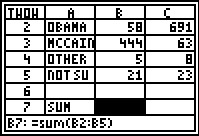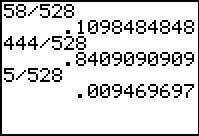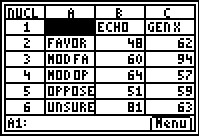# Activities

••• ##### Subject Area

• Math: Statistics: Data Analysis

• ##### Author9-12

20 Minutes

• ##### Device
• TI-83 Plus Family
• TI-84 Plus
• TI-84 Plus Silver Edition
•TI-84 Plus C Silver Edition
•TI-84 Plus CE
• ##### Software

TI Connect™
TI Connect™ CE

• ##### Report an Issue

It's a Two-Way Street#### Activity Overview

Students will be introduced to two-way tables by calculating marginal and conditional distributions using formulas in a spreadsheet.

#### Key Steps

•Students are given a two-way table and asked to calculate marginal distributions based on each category. They will use formulas to calculate sums and percentages. The teacher will follow up with discussion questions, asking students to interpret what the marginal distributions tell about the data.

•Students will then calculate conditional distributions for both the variables. They restrict their attention to each party affiliation and then restrict their attention to the candidate choice. Students are asked to interpret what the specific percentages in the table represent. The teacher document also has several discussion questions to further develop the understanding of how conditional distributions relate to the data.

•Students are given a problem that they can complete for homework. They are to use what they’ve learned in the activity to calculate the marginal and conditional distributions on their own.